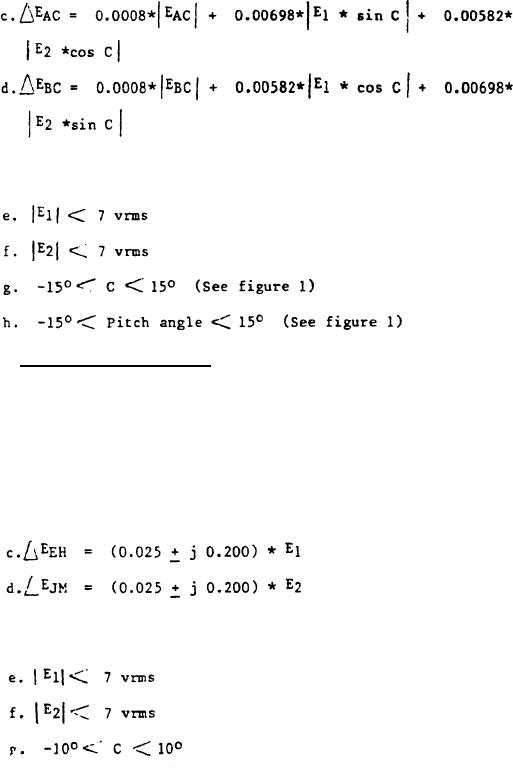Click here to make tpub.com your Home Page Page Title: Stator output voltages Back | Up | NextWeb www.tpub.comHome

Information Categories
Aerographer
Automotive
Aviation
Construction
Diving
Draftsman
Engineering
Electronics
Food and Cooking
Logistics
Math
Medical
Music
Nuclear Fundamentals
Photography
ReligionMIL-S-48461B(AR)
W h e r e C is the assembly angular displacement about the roll axis in degrees
( S e e figure 1)  T h e tolerance of the outputs shall be not greater than the
v a l u e of the following equations:
A p p l i c a t i o n of the transfer function and tolerance equations shall be valid
w i t h i n the following limits:
3 . 3 . 2 S t a t o r o u t p u t v o l t a g e s . T h e n o m i n a l o u t p u t v o l t a g e s  EE H a n d
E J M measured with respect to common shall be described by the following
transfer functions: (See 4.6.2.2)
a.
EEH =
- 1 . 0 2 6 * El
b.
EJ M =
-1.026 * E 2
T h e tolerance of the outputs shall be not greater than value of the following
equations:
A p p l i c a t i o n of the transfer function and tolerance equations shall be valid
w i t h i n the following limits:
6